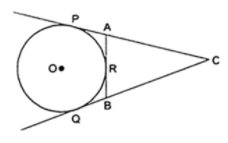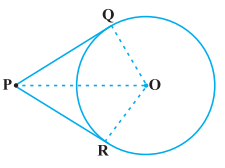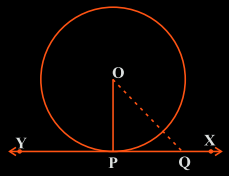QuestionAnswers

# In figure, CP and CQ are tangents to the circle with center O. ARB is another tangent touching the circle at R. If CP = 12 cm, and BC = 8 cm, then find the length of BR.Hint: Read the question carefully, and consider all the given information as it leads to solution.
Tangent to a circle is a line that intersects the circle at only one point.
The property of Pair of tangents to a circle drawn from an external point is the key to solve this question.

Complete step by step solution:
Step 1: Draw the labeled diagram carefully:Figure 1: circle with center O
Step 2: Given that:
Line CP, CQ, and AB are tangents to the circle with center O.
Length CP = 12 cm, BC = 8 cm
Step 3: State the theorem related to Pair of tangents to a circle drawn from an external point:
Theorem 1: The lengths of tangents drawn from an external point to a circle are equal.Figure 2: tangents PQ and PR to the circle with center O
Hence, length PQ = length PR
Step 4: apply the theorem
Here, line CP and CQ are tangents from external point C to the circle with center O.
$\Rightarrow$CP = CQ
Hence, CQ = 12 cm
Step 5: since, QBC is a straight line:
Therefore, CQ = BQ + BC
12 = BQ + 8
BQ = 12 – 8
$\Rightarrow$BQ = 4 cm. …… (1)
Step 6: apply the theorem
Here, line BQ and BR are tangents from external point B to the circle with center O.
$\Rightarrow$BQ = BR
Hence, BR = 4 cm (from (1))

Therefore, the required length of the BR is 4 cm.

Note:
Another important theorem of tangents and circle:
Theorem: The tangent at any point of a circle is perpendicular to the radius through the point of contact.Figure 3: tangent XY to the circle with center O.
Hence, $OP \bot PQ$
There is only one tangent at a point of the circle.
View Notes
CBSE Class 10 Maths Chapter 8 - Introduction to Trigonometry FormulaWhat are the Successor and Predecessor?Area of a Sector of a Circle FormulaWhat are the Functions of the Human Skeletal System?IMO Maths Olympiad Sample Question Paper for Class 6 to 10CBSE Class 8 Maths Chapter 15 - Introduction to Graphs FormulasCBSE Class 6 to 12 Maths Formulas - Important Math FormulasIMO Maths Olympiad Previous Year Question Paper for Class 5 to 10Group Theory in MathematicsTo Determine the Mass of Two Different Objects Using a Beam BalanceImportant Questions for CBSE Class 10 Maths Chapter 8 - Introduction to TrigonometryImportant Questions for CBSE Class 6 English A Pact with The Sun Chapter 9 - What Happened to The ReptilesImportant Questions for CBSE Class 10 Maths Chapter 12 - Areas Related to CirclesImportant Questions for CBSE Class 6 English A Pact with The Sun Chapter 6 - The Monkey and the CrocodileImportant Questions for CBSE Class 6 English A Pact with The Sun Chapter 10 - A Strange Wrestling MatchCBSE Class 7 Science Fibre to Fabric WorksheetsCBSE Class 6 Science Fibre to Fabric WorksheetsImportant Questions for CBSE Class 8 Maths Chapter 15 - Introduction to GraphsImportant Questions with Answers for CBSE Class 6 to 12 - All SubjectsImportant Questions for CBSE Class 6 English A Pact with The Sun Chapter 8 - A Pact with the SunCBSE Class 10 Hindi A Question Paper with SolutionsCBSE Previous Year Question Papers Class 10 Maths with SolutionsCBSE Class 10 Hindi A Question Paper 2020Hindi A Class 10 CBSE Question Paper 2009Hindi A Class 10 CBSE Question Paper 2015CBSE Class 10 English Language and Literature Question Paper with SolutionsCBSE Class 10 Maths Question Paper 2017CBSE Class 10 Maths Question Paper 2020Hindi A Class 10 CBSE Question Paper 2007Hindi A Class 10 CBSE Question Paper 2013RD Sharma Class 11 Maths Solutions Chapter 24 - The CircleRD Sharma Class 11 Solutions Chapter 24 - The Circle (Ex 24.2) Exercise 24.2RD Sharma Class 11 Solutions Chapter 24 - The Circle (Ex 24.1) Exercise 24.1NCERT Solutions for Class 10 Maths Chapter 8 Introduction to Trigonometry in HindiNCERT Solutions for Class 10 Maths Chapter 12 Areas Related to Circles in HindiRD Sharma Class 11 Solutions Chapter 24 - The Circle (Ex 24.3) Exercise 24.3NCERT Solutions for Class 10 Maths Chapter 8 Introduction to TrigonometryRS Aggarwal Class 11 Solutions Chapter-21 CircleNCERT Solutions for Class 10 Maths Chapter 12 Areas Related to CirclesRD Sharma Class 12 Maths Solutions Chapter 16 - Tangents and Normals